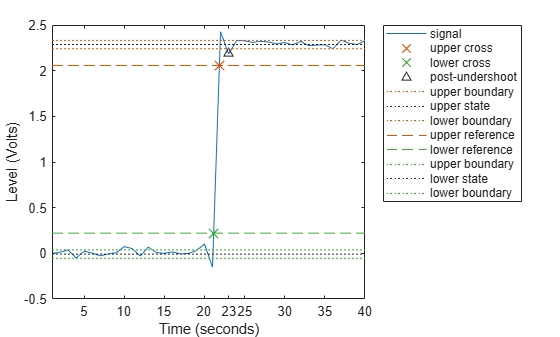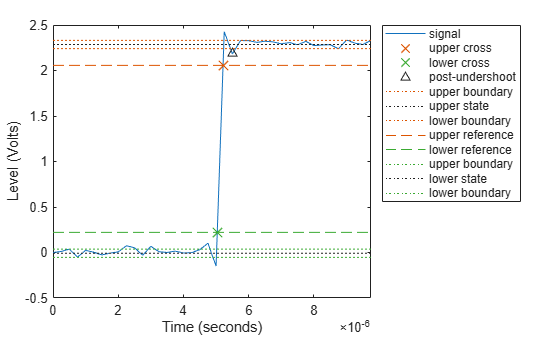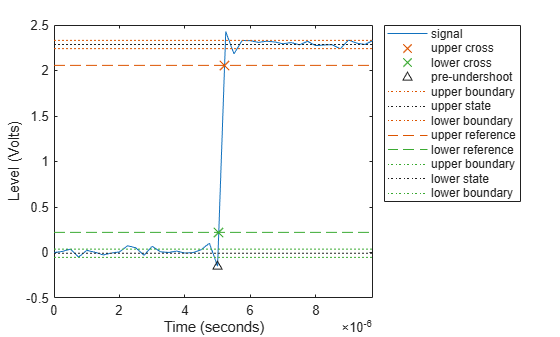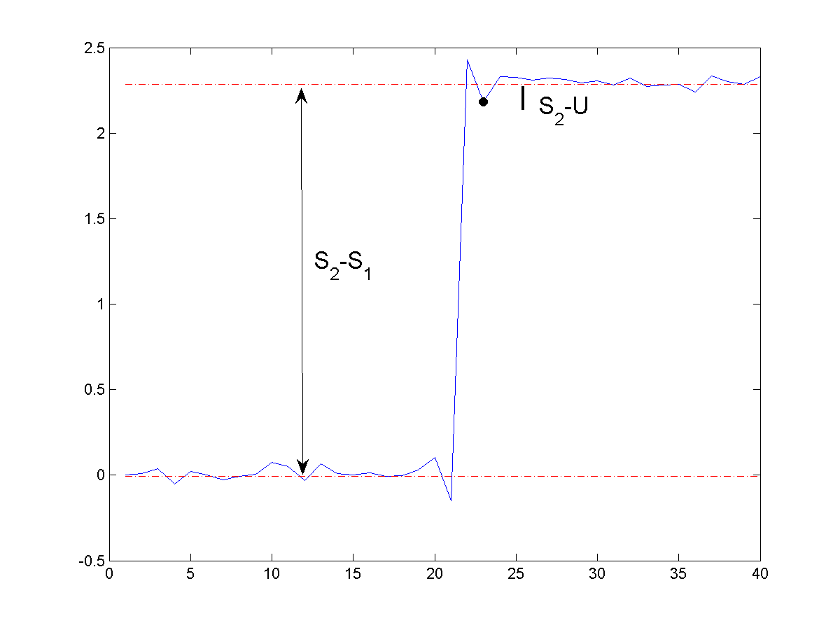Documentation

# undershoot

Undershoot metrics of bilevel waveform transitions

## Syntax

```US = undershoot(X) US = undershoot(X,FS) US = undershoot(X,T) [US,USLEV,USINST] = undershoot(...) [...] = undershoot(...,Name,Value) undershoot(...) ```

## Description

`US = undershoot(X)` returns the greatest deviations below the final state levels of each transition in the bilevel waveform, `X`. The undershoots, `US`, are expressed as a percentage of the difference between the state levels. See Undershoot. The length of `US` corresponds to the number of transitions detected in the input signal. The sample instants in `X` correspond to the vector indices. To determine the transitions, `undershoot` estimates the state levels of the input waveform by a histogram method. `undershoot` identifies all regions that cross the upper-state boundary of the low state and the lower-state boundary of the high state. The low-state and high-state boundaries are expressed as the state level plus or minus a multiple of the difference between the state levels. See State-Level Tolerances.

`US = undershoot(X,FS)` specifies the sample rate, `FS`, in hertz. The sample rate determines the sample instants corresponding to the elements in `X`. The first sample instant in `X` corresponds to t=0.

`US = undershoot(X,T)` specifies the sample instants, `T`, as a vector with the same number of elements as `X`.

```[US,USLEV,USINST] = undershoot(...)``` returns the levels, `USLEV`, and sample instants, `USINST`, of the undershoots for each transition.

`[...] = undershoot(...,Name,Value)` returns the greatest deviations below the final state level with additional options specified by one or more `Name,Value` pair arguments.

`undershoot(...)` plots the bilevel waveform and marks the location of the undershoot of each transition as well as the lower- and upper reference-level instants and the associated reference levels. `undershoot` also plots the state levels and associated lower- and upper-state boundaries.

## Input Arguments

 `X` Bilevel waveform. `X` is a real-valued row or column vector. `FS` Sample rate in hertz. `T` Vector of sample instants. The length of `T` must equal the length of the bilevel waveform, `X`.

### Name-Value Pair Arguments

 `'PercentReferenceLevels'` Reference levels as a percentage of the waveform amplitude. The lower-state level is defined to be 0 percent. The upper-state level is defined to be 100 percent. The value of `'PercentReferenceLevels'` is a 2-element real row vector whose elements correspond to the lower and upper percent reference levels. Default: `[10 90]` `'Region'` Specify the region over which to perform the undershoot computation. Valid values for `'Region'` are `'Preshoot'` or `'Postshoot'`. If you specify `'Preshoot'`, the end of the pretransition aberration region is defined as the last instant when the signal exits the first state. If you specify `'Postshoot'`, the start of the posttransition aberration region is defined as the instant when the signal enters the second state. Default: `'Postshoot'` `'SeekFactor'` Aberration region duration. Specifies the duration of the region over which to compute the undershoot for each transition as a multiple of the corresponding transition duration. The edge of the waveform may be reached, or a complete intervening transition may be detected, before the duration aberration region duration elapses. In such cases, the duration is truncated to the edge of the waveform or the start of the intervening transition. Default: `3` `'StateLevels'` Lower- and upper-state levels. Specify the levels to use for the lower- and upper-state levels as a 2-element real row vector whose first and second elements correspond to the lower- and upper-state levels of the input waveform. `'Tolerance'` Specify the tolerance that the initial and final levels of each transition must be within the respective state levels. The `'Tolerance'` value is a scalar expressing a percentage of the difference between the upper- and lower-state levels. See State-Level Tolerances. Default: `2`

## Output Arguments

 `US` Undershoots expressed as a percentage of the state levels. The undershoot percentages are computed based on the greatest deviation from the final state level in each transition. By default undershoots are computed for posttransition aberration regions. See Undershoot. `USLEV` Level of the pretransition or posttransition undershoot. `USINST` Sample instants of pretransition or posttransition undershoots. If you specify the sample rate or sampling instants, the undershoot instants are in seconds. If you do not specify the sample rate or sampling instants, the undershoot instants are the indices of the input vector.

## Examples

collapse all

Determine the maximum percent undershoot relative to the high-state level in a 2.3 V clock waveform.

Load the 2.3 V clock data. Determine the maximum percent undershoot of the transition. Determine also the level and sample instant of the undershoot. In this example, the maximum undershoot in the posttransition region occurs near index 23.

```load('transitionex.mat','x') [uu,lv,nst] = undershoot(x)```
```uu = 4.5012 ```
```lv = 2.1826 ```
```nst = 23 ```

Plot the waveform. Annotate the overshoot and the corresponding sample instant.

```undershoot(x); ax = gca; ax.XTick = sort([ax.XTick nst]);```Determine the maximum percent undershoot relative to the high-state level, the level of the undershoot, and the sample instant in a 2.3 V clock waveform.

Load the 2.3 V clock data with sampling instants. The clock data are sampled at 4 MHz.

`load('transitionex.mat','x','t')`

Determine the maximum percent undershoot, the level of the undershoot in volts, and the time instant where the maximum undershoot occurs. Plot the result.

`[us,uslev,usinst] = undershoot(x,t)`
```us = 4.5012 ```
```uslev = 2.1826 ```
```usinst = 5.5000e-06 ```
`undershoot(x,t);`Determine the maximum percent undershoot relative to the low-state level, the level of the undershoot, and the sample instant in a 2.3 V clock waveform. Specify the `'Region'` as `'Preshoot'` to output pretransition metrics.

Load the 2.3 V clock data with sampling instants. The clock data are sampled at 4 MHz.

`load('transitionex.mat','x','t')`

Determine the maximum percent undershoot, the level of the undershoot in volts, and the sampling instant where the maximum undershoot occurs. Plot the result.

`[us,uslev,usinst] = undershoot(x,t,'Region','Preshoot')`
```us = 6.1798 ```
```uslev = -0.1500 ```
```usinst = 5.0000e-06 ```
`undershoot(x,t,'Region','Preshoot');`## More About

collapse all

### Undershoot

For a positive-going (positive-polarity) pulse, undershoot expressed as a percentage is

`$100\frac{\left({S}_{2}-U\right)}{\left({S}_{2}-{S}_{1}\right)}$`

where U is the greatest deviation below the high-state level, S2 is the high state, and S1 is the low state.

For a negative-going (negative-polarity) pulse, undershoot expressed as a percentage is

`$100\frac{\left({S}_{1}-U\right)}{\left({S}_{2}-{S}_{1}\right)}$`

The following figure illustrates the calculation of undershoot for a positive-going transition.The red dashed lines indicate the estimated state levels. The double-sided black arrow depicts the difference between the high- and low-state levels. The solid black line indicates the difference between the high-state level and the undershoot value.

### State-Level Tolerances

Each state level can have associated lower- and upper-state boundaries. These state boundaries are defined as the state level plus or minus a scalar multiple of the difference between the high state and the low state. To provide a useful tolerance region, the scalar is typically a small number such as 2/100 or 3/100. In general, theregion for the low state is defined as

``

whereis the low-state level andis the high-state level. Replace the first term in the equation withto obtain thetolerance region for the high state.

The following figure illustrates lower and upper 2% state boundaries (tolerance regions) for a positive-polarity bilevel waveform. The red dashed lines indicate the estimated state levels.## References

 IEEE® Standard on Transitions, Pulses, and Related Waveforms, IEEE Standard 181, 2003, pp. 15–17.